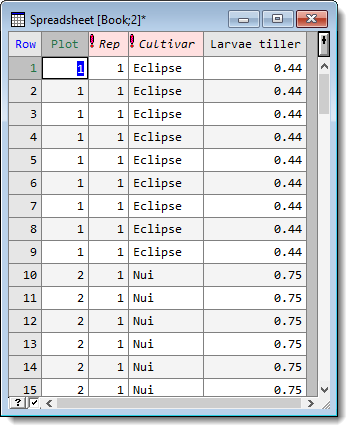1. Home
2. Expanding a Spreadsheet Using a Weight Column

# Expanding a Spreadsheet Using a Weight Column

Most Genstat analyses have an option to add weights for an analysis. For those Genstat analyses that don’t have a weights option you can still do a weighted analysis by expanding the spreadsheet to duplicate each row the number of times given by the weights. You can then use the expanded spreadsheet to perform the equivalent of the weighted analysis.

In the following spreadsheet image a number of ryegrass tillers was sampled in each plot and the number of weevil larvae in these tillers was counted. The column Larvae tiller contains the mean number of larvae per tiller.If we want to use the number of tillers counted as weights in an analysis that doesn’t allow for weights, we can expand the spreadsheet using the Tillers column as weights. Using this data would be equivalent to specifying the Tillers column as weights.

1. Select Spread | Manipulate | Expand.2. Select the column that contains the weights to be used in the expansion.
3. Leave options at their defaults or set them as required then click OK.If values within the weight column are not integers the values will be rounded to the nearest integer. If a weight value is less than zero or a missing value then no copies of that row will appear in the new spreadsheet. If a weight value is zero, then rows will only be copied if the Keep rows with zero weight option is selected.

The CALCULATE function NEXPAND can be used to expand a data column in command mode. However, this function can only be used with numerical columns, and factors are converted to variates.

The menu will produce a warning if the weights seem excessively large. The new spreadsheet will not contain the original weights column.

Options